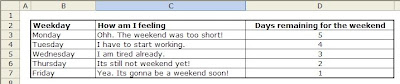### How to use VLookUp in Microsoft Excel.

Tool : MS Excel.
Topic : The function - VLOOKUP.

This is a very useful function to extract data from the table.

Microsoft says..
"Searches for a value in the leftmost column of a table, and then returns a value in the same row from a column you specify in the table."

The format.
VLOOKUP(lookup_value, table_array, col_index_num, range_lookup)

where..
lookup_value = search key for the first column.
table_array = Table where the data is located.
col_index_num = is the column where the data has to be extracted from.
range_lookup = TRUE or omited then finds an approximate match. FALSE then finds an exact match and returns #N/A if there is no match.

Lets take an example. Let the data table be the following one.From this table, you can find how are you feeling [ :) ] and how many days are left for the next weekend [ :)) ].
it will look something like this..if you replace TUESDAY with another valve, the other two values will get extracted from the table.

How it works.
the formula for the cell C11 is this.
=VLOOKUP(\$C\$10,\$B\$3:\$D\$7,2,0)

the formula for the cell C12 is this.
=VLOOKUP(\$C\$10,\$B\$3:\$D\$7,3,0)

where,

C10 is the feed data or the data you want to search in the first column.
B3:D7 is the table shown in the first picture. (the source data)
next is column where you want the data from. Its 2 and 3 respectively,
next is 0 [ equal to false ]

Note (1):
You can ignore the \$ symbols if its complicated.
\$ sign is to lock the movement of the reference cell when the cell is copied to another one.

Note (2):
You can define a name for the table which can be used in functions.
Insert -> Name -> Define.
Put the name as "Data_Table"
Put the value as "B2:D7"

So the formulae will change as
=VLOOKUP(\$C\$10,Data_Table,2,0)
=VLOOKUP(\$C\$10,Data_Table,3,0)

Hope this is a good introduction for you to start exploring it further yourself.
put a comment if there is anything you want to let me know.Anonymous said…
Its lovely.
Do you have an Excel function/s that searchs a table for MAX (or MIN), find it and then returns the Cell Index.
Ex lets have a table A1:F10 .
lets say that in this specific table the MAX value is at cell C8.
so the function has to return the value C8.

### TreeView Structure in Excel VBA

TreeView is extreamly useful in specific cases but it can be bit tricky sometimes to implement.  Last Few days I was working on a TreeView Structure and thought I will share the knowledge I gained...  This post takes you through the basic operations to create and operate a TreeView. It will be like the Folder tree window of the windows explorer.  Moreover, you can have it dynamically updated based on the data in excel.... Here we go..

### Controlling LED through Octolapse for 3D print timelapse

Today's post is about one of the 3D Printing adaptation that I learned. This is specifically about how to control an LED light automatically through OctoLapse so that it is switched on only when the timelapse photo is taken. Just as a background, I have been learning to use 3D printer and its customizations options for a few months now and was a lot of learning since then. The best thing was OctoPi, a platform for controlling the 3D printer and the many plugin options that are developed by the community.  Most of them are like open source. Today we will discuss specifically about an adaptation to one such plugin, OctoLapse. OctoLapse is the plugin for taking timelapse photos, with many options for further customisations. One of the best timelapse method is taking photo after each layer is complete, by moving the head out of the way. You can use standard Pi Camera or a DSLR, which is great to get good resolution videos. One small flash back before we dive into the topic.  I keep the